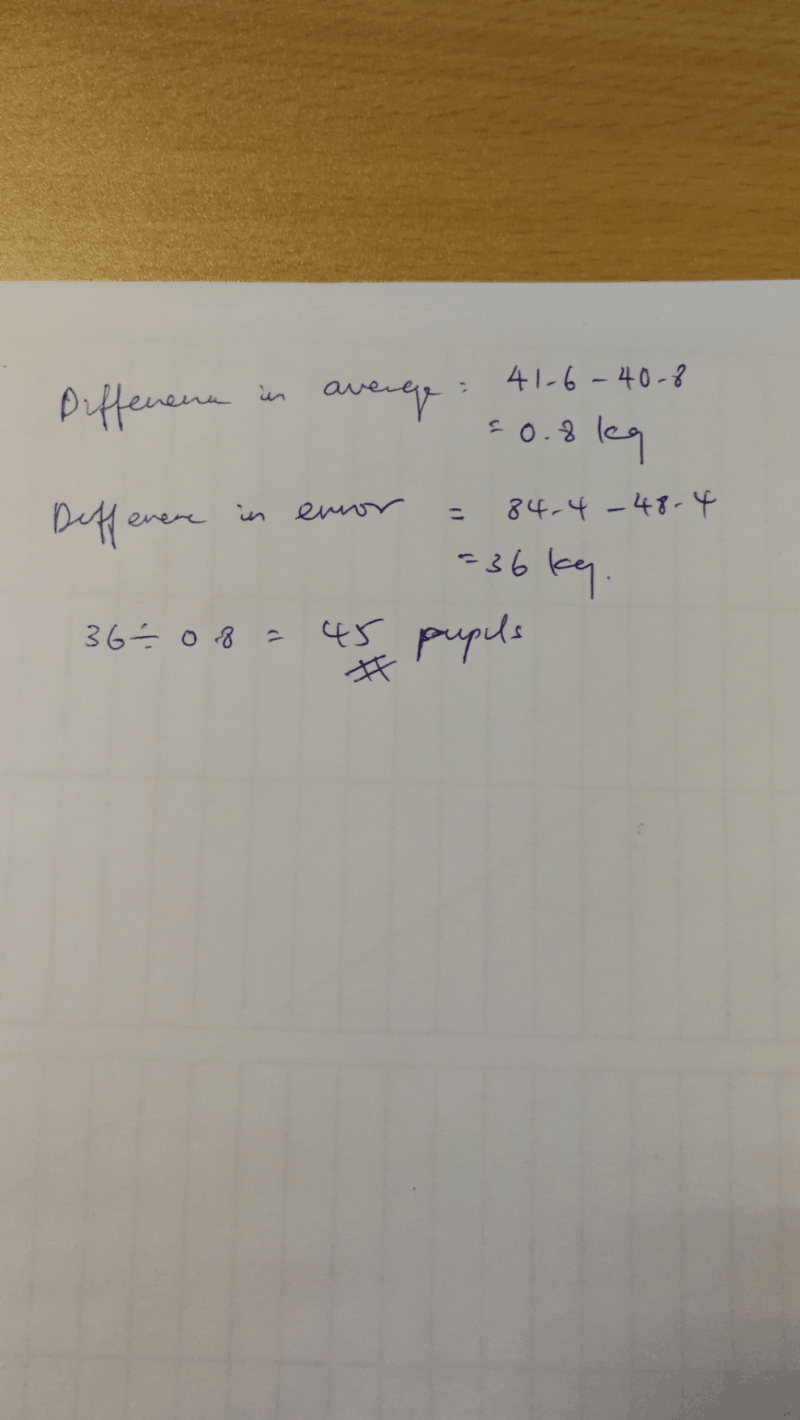# Question

The average mass of a group of pupils was 41.6kg.  The mass of one of the pupils was wrongly recorded as 48.4 kg, when it should have been 84.4 kg.  As a result, the average mass of the pupils was wrongly calculated as 40.8 kg.  How many pupils are there in the group?Hi,

Can you kindly explain why that the difference and divide.

Thank you!